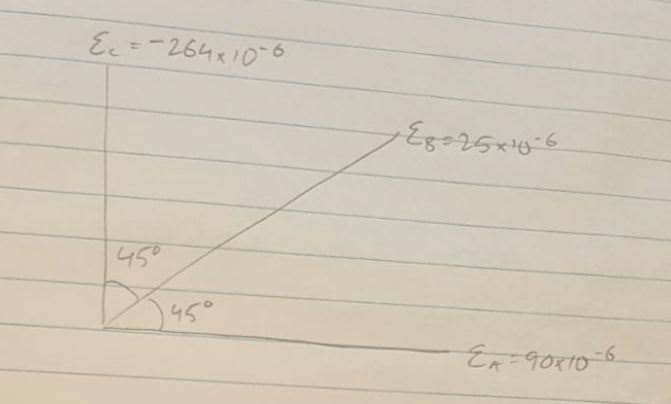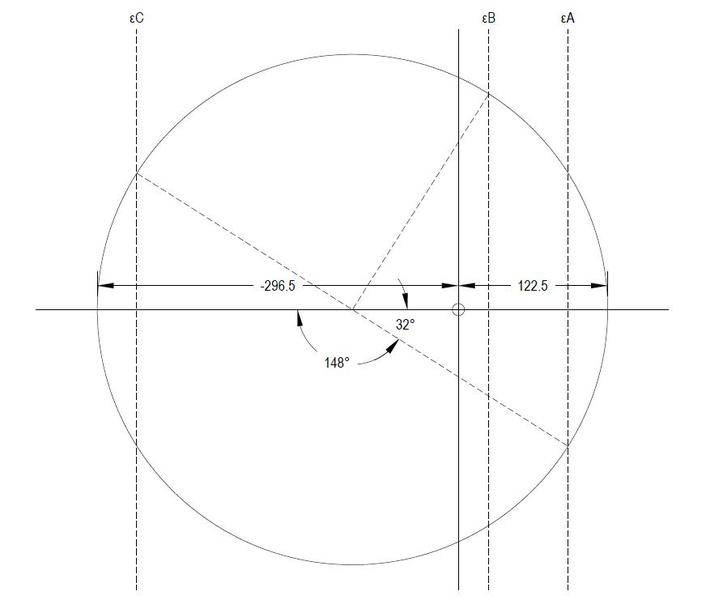# Creating Mohr's strain circle from a strain rosette

• ridiculoid

## Homework Statement

A sample is put in tension and a strain rosette gives the following results:i) Calculate principal strains and poissons ratio using Mohrs strain circle.
ii) Calculate principal stresses from principal strains and poissons ratio.

## Homework Equations

Mohr's strain circle
γ = -lateral strain / axial strain
σx = E / 1 - γ2 (εx + γεy)
σy = E / 1 - γ2 (εy + γεx)
E = 210 GPa

## The Attempt at a Solution

I have drawn up Mohr's circle in autocad and found the following results.principal strains = 122.5x10-6 & -296.5x10-6

poissons ratio = -122.5 / -296.5 = 0.413 (this seems too high?)

principal stresses = σx = 11.5kPa (this is supposed to be low as the load is applied in the y direction?)
σy = -62.99MPa (should this be negative if its in tension?)

Im hoping someone can tell me if I am on the right track.

#### Attachments

Is this supposed to be plane stress or plane strain?

## Homework Statement

A sample is put in tension and a strain rosette gives the following results:
View attachment 231132
i) Calculate principal strains and poissons ratio using Mohrs strain circle.
ii) Calculate principal stresses from principal strains and poissons ratio.

## Homework Equations

Mohr's strain circle
γ = -lateral strain / axial strain
σx = E / 1 - γ2 (εx + γεy)
σy = E / 1 - γ2 (εy + γεx)
E = 210 GPa

## The Attempt at a Solution

I have drawn up Mohr's circle in autocad and found the following results.
View attachment 231133
principal strains = 122.5x10-6 & -296.5x10-6

poissons ratio = -122.5 / -296.5 = 0.413 (this seems too high?)

principal stresses = σx = 11.5kPa (this is supposed to be low as the load is applied in the y direction?)
σy = -62.99MPa (should this be negative if its in tension?)

Im hoping someone can tell me if I am on the right track.